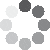Enter parameters LENGTH, WIDTH & DEPTH
Enter parameters
LENGTH, WIDTH & DEPTH
Length:
ft
• inches (in)
• feet (ft)
• yards (yd)
• centimeters (cm)
• meters (m)
Width:
ft
• inches (in)
• feet (ft)
• yards (yd)
• centimeters (cm)
• meters (m)
Depth:
in
• inches (in)
• feet (ft)
• yards (yd)
• centimeters (cm)
• meters (m)
Density:
140 - Asphalt lb/ft³
• Asphalt - 140 lb/ft³
• Custom
Price per unit of mass:
t
• pound (lb)
• US short ton (t)
• imperial-long ton (long t)
• kilogram (kg)
Price per unit of volume:
yd³
• cubic yards (yd³)
• cubic feet (ft³)
• cubic inches (in³)
• cubic centimeters (cm³)
• cubic meters ()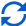Result
Area:
0
ft²
• square feet (ft²)
• square inches (in²)
• square yards (yd²)
• square miles (mi²)
• acres (ac)
• square centimeters (cm²)
• square meters ()
Volume:
0
yd³
• cubic yards (yd³)
• cubic feet (ft³)
• cubic inches (in³)
• cubic centimeters (cm³)
• cubic meters ()
Weight:
0
t
• pounds (lb)
• US short tons(t)
• imperial-long tons (long t)
• kilograms (kg)
Cost:
$Enter parameters AREA & DEPTH Area: ft² • square feet (ft²) • square inches (in²) • square yards (yd²) • square miles (mi²) • acres (ac) • square centimeters (cm²) • square meters () Depth: in • inches (in) • feet (ft) • yards (yd) • centimeters (cm) • meters (m) Density: 140 - Asphalt lb/ft³ • Asphalt - 140 lb/ft³ • Custom Price per unit of mass: t • pounds (lb) • US short tons (t) • imperial-long tons (long t) • kilograms (kg) Price per unit of volume: yd³ • cubic yards (yd³) • cubic feet (ft³) • cubic inches (in³) • cubic centimeters (cm³) • cubic meters ()Result Volume: 0 yd³ • cubic yards (yd³) • cubic feet (ft³) • cubic inches (in³) • cubic centimeters (cm³) • cubic meters () Weight: 0 t • pounds (lb) • US short tons (t) • imperial-long tons (long t) • kilograms (kg) Cost:$
Enter parameters
VOLUME
Volume:
yd³
• cubic yards (yd³)
• cubic feet (ft³)
• cubic inches (in³)
• cubic centimeters (cm³)
• cubic meters ()
Density:
140 - Asphalt lb/ft³
• Asphalt - 140 lb/ft³
• Custom
Price per unit of mass:
t
• pounds (lb)
• US short tons (t)
• imperial-long tons (long t)
• kilograms (kg)
Price per unit of volume:
yd³
• cubic yards (yd³)
• cubic feet (ft³)
• cubic inches (in³)
• cubic centimeters (cm³)
• cubic meters ()Result
Weight:
0
t
• pounds (lb)
• US short tons (t)
• imperial-long tons (long t)
• kilograms (kg)
Cost:
$(No Ratings Yet)Loading... Similar Calculators: Real-time graphics. Make the calculations and see the changes. Real-time graphics. Make the calculations and see the changes. Real-time graphics. Make the calculations and see the changes. Embed Similar Calculators:We know that working out how much asphalt is needed to resurface part of a road is not easy. Estimating by eye is even more tricky! You want to be as accurate as possible to save you money and time. That’s why we think our online asphalt calculator is an excellent aide. 👍 Keep reading below to see how to use it, what it does and how it can help you calculate the total volume, weight and cost of asphalt. Using it will help you be more effective and efficient with your next project! Now, without further ado… Contents: ## Asphalt calculator formula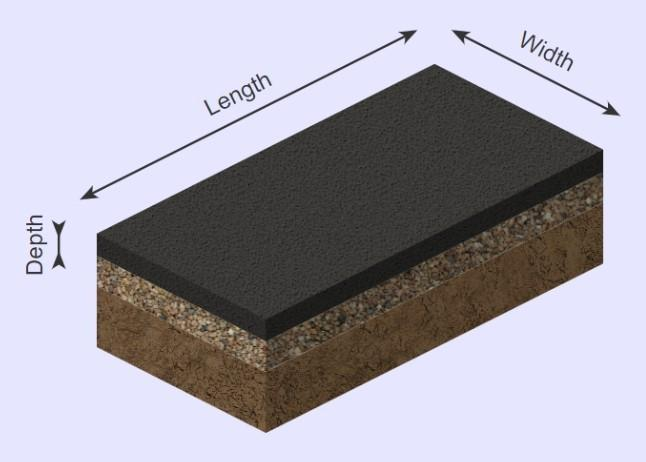Based on the measurements you provide for required length, width and thickness, the calculator first works out the area and volume of the hot asphalt mix required using the below formulas: $$Area=Length \times Width$$ $$Volume = Area \times Depth$$ In addition, if you know the density of the asphalt, it can calculate how many tons are required: $$Weight = Volume \times Density$$ NB: Standard asphalt is 140 pounds per cubic foot. But wait, there’s more! If you know the cost of the asphalt per unit volume or mass, the calculator will also work out the total cost for you: $$Cost = Price\,per\,unit\,mass \times Weight$$ or $$Cost = Price\,per\,unit\,volume \times Volume$$ If you don’t know the price per unit mass/volume, calculate it using the formulas: $$Price\,per\,unit\,mass = {Total\,cost\,of\,asphalt \over Weight\,of\,asphalt}$$ or $$Price\,per\,unit\,volume = {Total\,cost\,of\,asphalt \over Volume\,of\,asphalt}$$ Something isn’t clear? 🤔 Continue reading our article to see three full examples with different paving requirements. Otherwise, go ahead and enter your measurements to get your landscaping project started off quickly and efficiently! ## Calculation examples • Paving standard asphalt Imagine I want to pave over an area measuring 20 feet by 4 feet to a thickness of 6 inches using standard asphalt (140 lb/ft³) and costing$150 per US short ton.

I want to have an estimate for the volume of asphalt required, along with its weight in tons and total cost.

The calculator would perform the following estimations to work out the weight of the asphalt:

$$Area = Length \times Width = 4\,ft \times 20\,ft = 80\,ft^2$$

$$Volume = Area \times Depth = 80\,ft^2 \times 6\,in = 40\,ft^3$$

$$Weight = Volume \times Density = 40\,ft^3 \times 140\,lb/ft^3 = 2.8\,t$$

$$Cost = Price\,per\,unit\,mass \times Weight = 150\,/t \times 2.8\,t = 420$$

But…

What if you don’t know the unit price for mass/volume?

• Pave 300 square feet calculating price per unit mass

Let’s imagine I have a path measuring 300 square feet (for example, 150ft x 2ft) and I want to asphalt this path to a thickness of 8 inches.

I am going to use a standard hot mix asphalt. I am not sure of its cost per unit mass/volume, but I can buy 18 tons of asphalt for a cost of $2160. I can therefore calculate the price per unit mass using the formula: $$Price\,per\,unit\,mass = {Total\,cost\,of\,asphalt \over Weight\,of\,asphalt} = {2160 \over 18\,t } = 120\,/t$$ At this point, I am now able to enter the values into the asphalt volume calculator. The estimator does the following calculations to work out the volume in cubic yards, weight in tons and cost in dollars. $$Volume = Area \times Depth = 300\,ft^2 \times 8\,in=7.407\,yd^3$$ $$Weight = Volume \times Density = 7.407\,yd^3 \times 140\,lb/ft^3 = 14\,t$$ $$Cost = Price\,per\,unit\,mass \times Weight = 120\,/t \times 14\,t = 1680$$ Finally: What if you don’t know the density of your asphalt or you are using a different type of aggregate than 140 lb/ft³ asphalt? Well, in the density option you can enter any custom density value, which can be calculated using the following formula: $$Density = {Mass \over Volume}$$ In this last example we will show you how to use this. • Cover 300 cubic feet of driveway with custom aggregate In our final example, let’s imagine we want to asphalt a total volume of 300 cubic feet of pathway with a custom aggregate costing$100 per ton.

I am not sure of the density, but it weighs 4,800 pounds per 60 cubic feet.

I can therefore calculate its density:

$$Density = {Mass \over Volume} = {4,800\,lb \over 60\,ft^3} =80\,lb/ft^3$$

Finally, I enter the measurements as shown to calculate the total weight and cost of the paving aggregate:

$$Weight = Volume \times Density = 300\,ft^3 \times 80\,lb/ft^3 = 12\,t$$

$$Cost = Price\,per\,unit\,mass \times Weight = 100\,/t \times 12\,t = 1200$$

You’ve likely noticed us using the units interchangeably; for example, in our second example density is given in pounds per cubic feet and volume is in cubic yards.

## What if my measurements are in different units?

The best thing about our asphalt calculator is that it takes care of this for you.

There are many options for each measurement – for example, length can be in inches (in), feet (ft), yards (yd), centimeters (cm) or meters (m).

All you need to do is select the required units in the options and the calculator will perform the following conversions:

$$1\,foot = 12\,inches = 0.33\,yards = 30.48\,centimeters = 0.3048\,meters$$

$$1\,US\,short\,ton = 2000\,pounds = 0.893\,imperial\,long\,ton = 907\,kg$$

Finally, you might wonder what you should do if your path is a combination of areas, like in our example.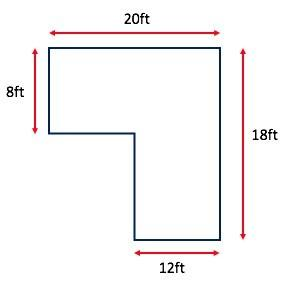The first step is to divide the path into squares or rectangles (or another basic shape whose area is easy to calculate).

Then work out the area of each of the squares or rectangles – you may need to work out some additional lengths like we have in our example.

This is easily done by adding or subtracting the appropriate lengths to find the dimensions of the squares or rectangles.

Finally, add the areas (or subtract if appropriate!) to calculate the total path area in square feet, yards, centimetres, etc.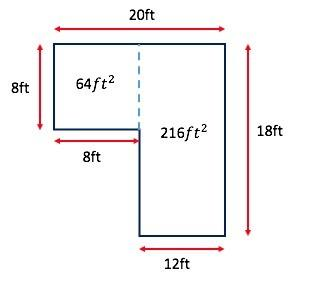In our example:

$$64\,ft^2 + 216\,ft^2 = 280\,ft^2$$

It’s that simple! 😎

Embed this calculator on your site!Add live graphics
Copied to clipboard! Preview